## Sinusoidal Response of RLC Circuit:

Consider a Sinusoidal Response of RLC Circuit consisting of resistance, inductance and capacitance in series as shown in Fig. 12.20.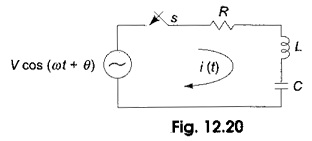Switch S is closed at t = 0. At t= 0, a sinusoidal voltage V cos (ωt + θ) is applied to the RLC series circuit, where V is the amplitude of the wave and θ is the phase angle. Application of Kirchhoff s voltage law to the Sinusoidal Response of RLC Circuit results in the following differential equation.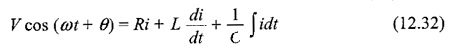Differentiating the above equation, we get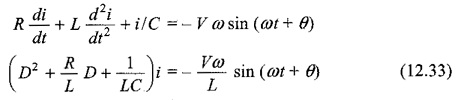The particular solution can be obtained by using undetermined coefficients. By assuming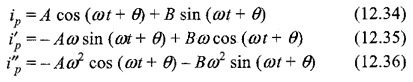Substituting ip, i′p and i″p in Eq. 12.33, we have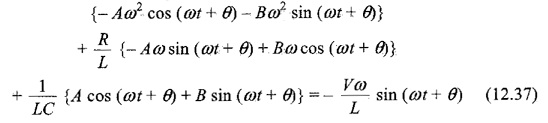Comparing both sides, we have

Sine coefficients.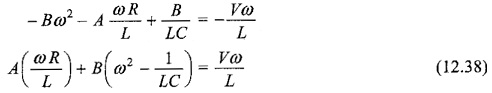Cosine coefficients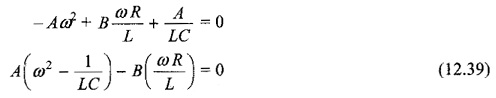Solving Eqs 12.38 and 12.39, we get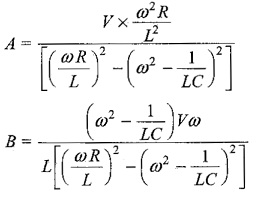Substituting the values of A and B in Eq. 12.34, we get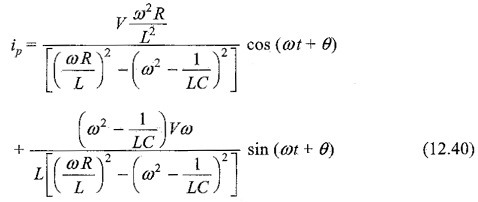Putting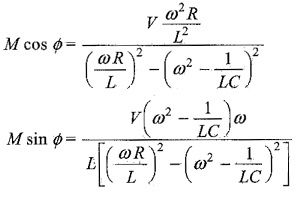To find M and Φ we divide one equation by the other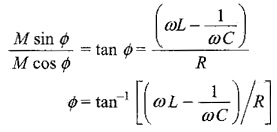Squaring both equations and adding, we get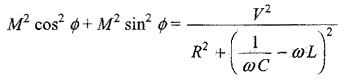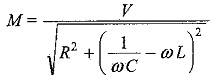The particular current becomes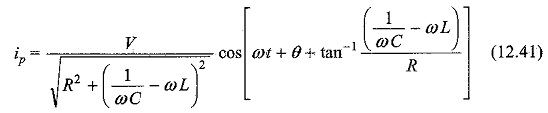The complementary function is similar to that of DC series RLC circuit. To find out the complementary function, we have the characteristic equation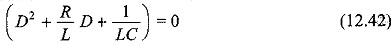The roots of Eq. 12.42, are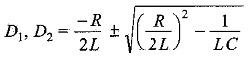By assuming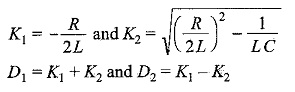K2 becomes positive, when (R/2L)2 > 1/LC

The roots are real and unequal, which gives an overdamped response. Then Eq. 12.42 becomes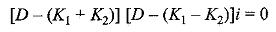The complementary function for the above equation is

ic = c1 e(K1 + K2)t  + c2 e(K1 – K2)t

Therefore, the complete solution is

i = ic + ip

= c1 e(K1 + K2)t  + c2 e(K1 – K2)t

K2 becomes negative, when (R/2L)2 < 1/LC

Then the roots are complex conjugate, which gives an underdamped response. Equation 12.42 becomes

[D – (K1 + jK2)] [D – (K1 – jK2)]i = 0

The solution for the above equation is

ic = eK1t [c1 cos K2t + c2 sin K2t]

Therefore, the complete solution is

i = ic + ip

i = eK1t [c1 cos K2t + c2 sin K2t]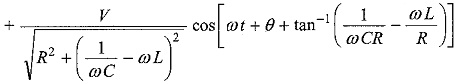K2 becomes zero, when (R/2L)2 = 1/LC

Then the roots are equal which gives critically damped response. Then, Eq. 12.42 becomes (D – K1) (D – K1) i = 0.

The complementary function for the above equation is

ic = eK1t (c1 + c2t)

Therefore, the complete solution is

i = ic + ip

i = eK1t (c1 + c2t)

Scroll to Top